# Voynich Word Order

2017-04-19

I've investigated whether the word order in the Voynich Manuscript is random, or more specifically, whether or not it's consistent with words being generated solely according to their probability within their page cluster or section.

Rene Zandbergen suggested that this could be checked by measuring the frequency of repeating words, and comparing it with the frequency that would be expected by chance. As it's not much more effort to measure the frequency of word pairs in general, I decided to do that instead.

Although the actual number of word pairs can be counted without dividing the text into page clusters, the expected numbers should calculated per page cluster and the result summed. Within any page cluster k, the probability of a word pair wi, wj is simply the product of each of the two words in k considered individually:

pk(wiwj) = pk(wi)pk(wj)

This is independent of any words already output, i.e. each word has a fixed probability, not a fixed count. So, for example, if an insufficient number of `daiin` were output before nearing the end of the page cluster, it would not result in a substantially raised frequency of `daiin` then being output to compensate, as it would be were the words picked out of a bag instead of output randomly.

The exact probabilities are unknown and can only be estimated from the word counts in the individual page clusters, i.e. for cluster k

pk(wi) = nk(wi)/Nk

i.e. the probability of a word i is its number of occurrences divided by the total number of words in the cluster. So the expected number of word pairs wi, wj in k is

ek(wiwj) = Nkpk(wi)pk(wj) = nk(wi)nk(wj)/Nk

and the total is therefore

Σknk(wi)nk(wj)/Nk

If words are output randomly, the measured pair count, plotted against its expected value should show points scattered about a line of gradient 1 passing through the origin.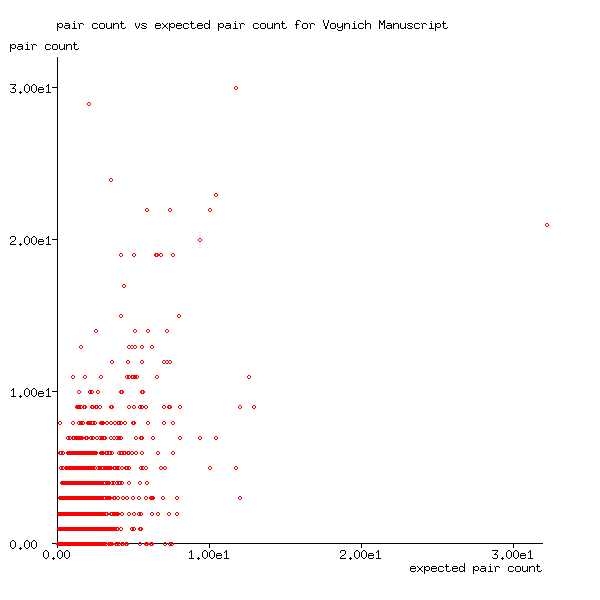Figure 1: Pair count plotted against expected pair count for Voynich Manuscript

In Figure 1, it's difficult to "see the wood from the trees", because many different word pairs have similar expected numbers, but whose actual numbers fluctuate around a mean value. Because there's a lower bound of 0 and no upper bound, and identical values overwrite one another, Figure 1 appears to have quite a steep gradient, instead of the gradient of 1.0 that one would expect. But when the mean of their numbers is plotted against the expected number instead, as in Figure 2, the results do clearly show a gradient of approximately one for values with many word pairs. The few outliers are those with few word pairs, i.e. those with high values of expected numbers. Because there are so few, their mean values are less stable. (For example, the rightmost word in the plot is the single word pair `daiin daiin`).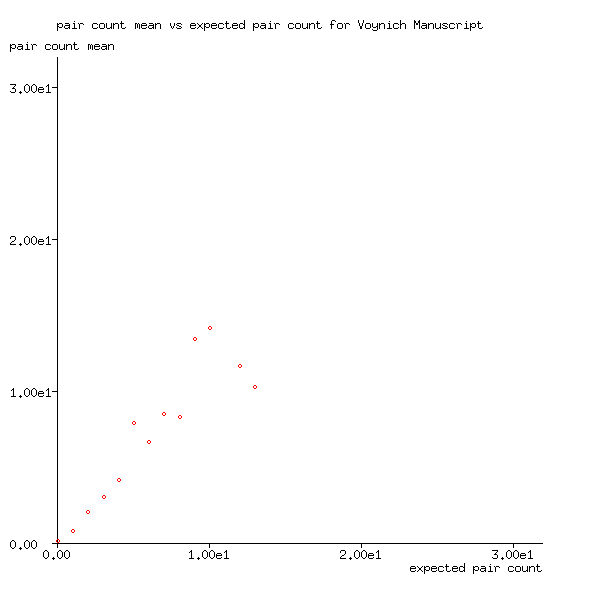Figure 2: Pair count mean plotted against expected pair count for Voynich Manuscript

Figure 2 strongly suggests that words in the Voynich Manuscript were randomly output onto the page, i.e. with no underlying order. This result is in disagreement with Probing the Statistical Properties of Unknown Texts: Application to the Voynich Manuscript by Amancio et al.

As words in the generated Voynich Manuscript are known to be output randomly, it should come as no surprise that the mean number of word pairs is close to its expected value: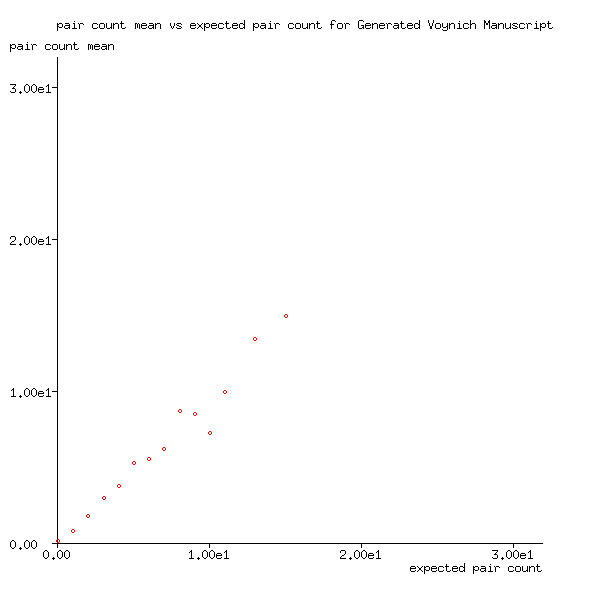Figure 3: Pair count mean plotted against expected pair count for generated Voynich Manuscript

Also, for the generated manuscript, Figure 4 shows that the variance of the number of word pairs is very close to expected pair count, which Figure 3 has shown is very close to the mean value, at least for values calculated from many word pair counts. In other words, the pair counts follow a Poisson distribution.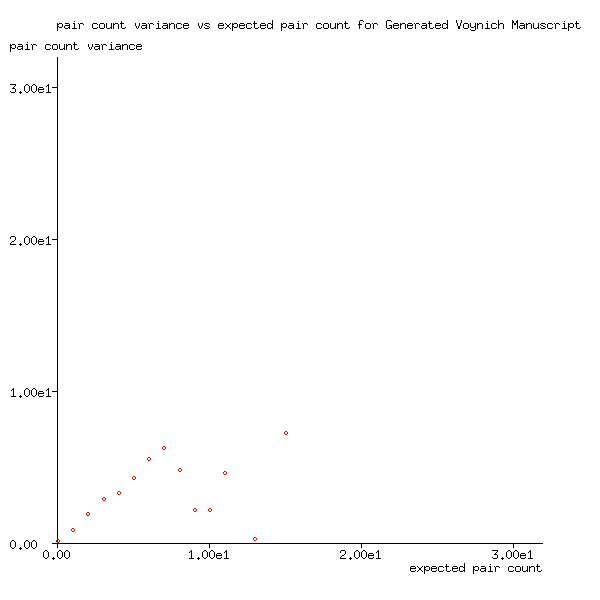Figure 4: Pair count variance plotted against expected pair count for the generated Voynich Manuscript

However, instead of following the Poisson distribution, Figure 5 shows that the pair counts of the Voynich Manuscript follow the Gamma distribution, for which variance = mean2 or, equivalently, standard deviation = mean, rather than variance = mean. Although it still suggests that the Voynich Manuscript's words are generated randomly, the word pair counts are significantly less predictable than those of my generated text.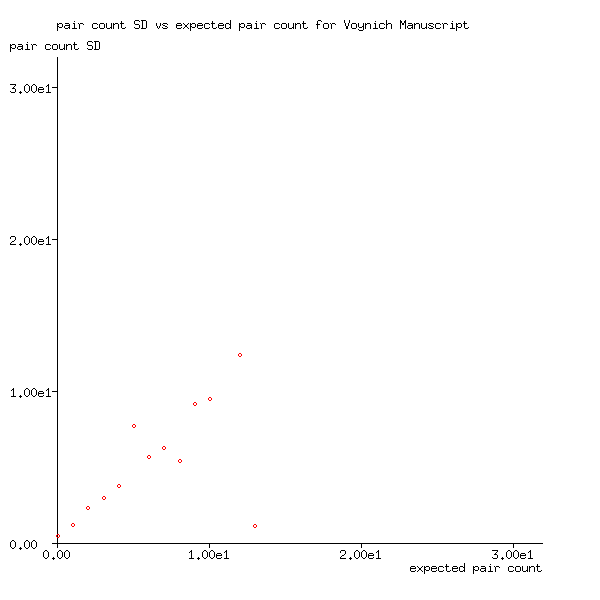Figure 5: Pair count standard deviation plotted against expected pair count for the Voynich Manuscript

At present, I have no explanation of this, but one possibility is that the random decision-making process used to generate the Voynich Manuscript differed significantly from the uniform random process used in my model, which is equivalent to drawing cards from a shuffled deck. A Poisson process might work better, but they take time to run.

## This just in

In An explanation of the Voynich Manuscript text #44, Torsten Timm pointed out that words ending with `y` are more frequently followed by words beginning with `qo` than you'd expect by chance (see Papers on the Voynich Manuscript). Figure 6 shows that this is indeed the case, as the gradient is significantly higher than 1.0.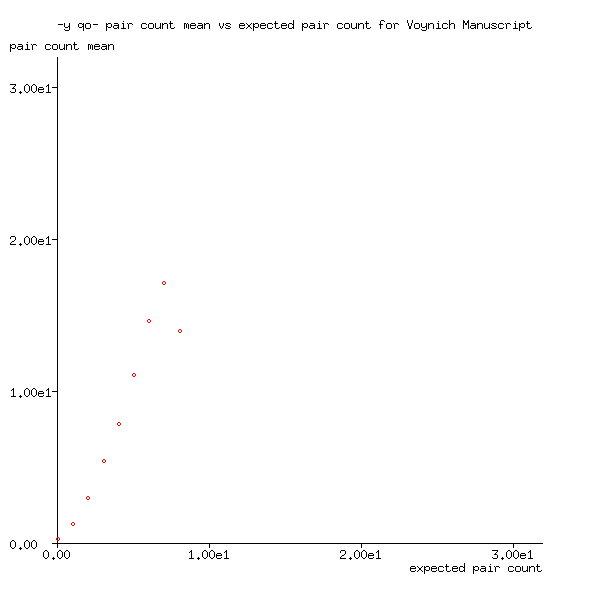Figure 6: Pair count mean for -y qo- plotted against expected pair count for Voynich Manuscript

If it were just an individual word, I'd discount it, but it's a whole class of words, so it forces a rethink. One approach to tackling it is to have a transition from final states back to initial states, either directly or through different space states.

Up